# A bar magnet is passingQuestion:

A bar magnet is passing through a conducting loop of radius $R$ with velocity $v$. The radius of the bar magnet is such that it just passes through the loop. The induced e.m.f. in the loop can be represented by the approximate curve :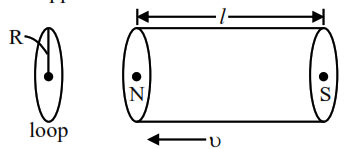1.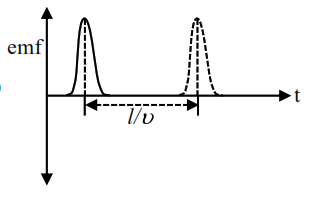2.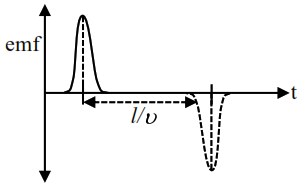3.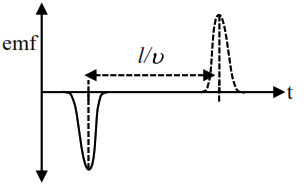4.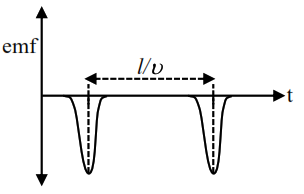Correct Option: , 3

Solution: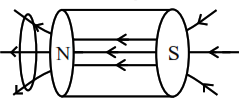$\rightarrow$ When magnet passes through centre region of solenoid, no current / Emf is induced in loop.

$\rightarrow$ While entering flux increases so negative induced emf

$\rightarrow$ While leaving flux decreases so positive induced emf.